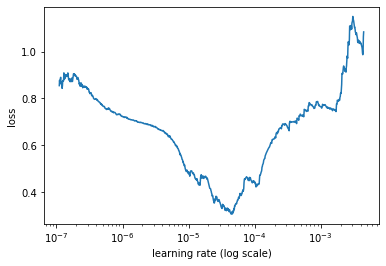In :
%reload_ext autoreload
%matplotlib inline
import os
os.environ["CUDA_DEVICE_ORDER"]="PCI_BUS_ID";
os.environ["CUDA_VISIBLE_DEVICES"]="0";

In :
import ktrain
from ktrain import text

In :
trn, val, preproc = text.texts_from_folder('data/aclImdb',
maxlen=500,
preprocess_mode='bert',
train_test_names=['train',
'test'],
classes=['pos', 'neg'])

detected encoding: utf-8
preprocessing train...
language: en

done.
Is Multi-Label? False
preprocessing test...
language: en

done.
In :
model = text.text_classifier('bert', trn , preproc=preproc)

Is Multi-Label? False
maxlen is 500
done.

In :
learner = ktrain.get_learner(model,
train_data=trn,
val_data=val,
batch_size=6)

In :
learner.lr_find()

simulating training for different learning rates... this may take a few moments...
Epoch 1/1024
6492/25000 [======>.......................] - ETA: 19:19 - loss: 0.6908 - acc: 0.6155

done.
Please invoke the Learner.lr_plot() method to visually inspect the loss plot to help identify the maximal learning rate associated with falling loss.

In :
learner.lr_plot()In :
# 2e-5 is one of the LRs  recommended by Google and is consistent with the plot above.
learner.fit_onecycle(2e-5, 1)

begin training using onecycle policy with max lr of 2e-05...
Train on 25000 samples, validate on 25000 samples
25000/25000 [==============================] - 2304s 92ms/sample - loss: 0.2442 - accuracy: 0.9008 - val_loss: 0.1596 - val_accuracy: 0.9394

Out:
<tensorflow.python.keras.callbacks.History at 0x7f6b102fe780>

### 93.94% accuracy in a single epoch.¶

Let's make some predictions on new data.

In :
predictor = ktrain.get_predictor(learner.model, preproc)

In :
data = [ 'This movie was horrible! The plot was boring. Acting was okay, though.',
'The film really sucked. I want my money back.',
'The plot had too many holes.',
'What a beautiful romantic comedy. 10/10 would see again!',
]

In :
predictor.predict(data)

Out:
['neg', 'neg', 'neg', 'pos']

To save and reload the the predictor for later use:

predictor.save('/tmp/my_predictor')